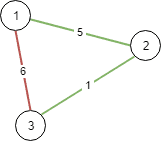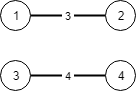# Question

Formatted question description: https://leetcode.ca/all/1135.html

There are n cities labeled from 1 to n. You are given the integer n and an array connections where connections[i] = [xi, yi, costi] indicates that the cost of connecting city xi and city yi (bidirectional connection) is costi.

Return the minimum cost to connect all the n cities such that there is at least one path between each pair of cities. If it is impossible to connect all the n cities, return -1,

The cost is the sum of the connections' costs used.

Example 1:Input: n = 3, connections = [[1,2,5],[1,3,6],[2,3,1]]
Output: 6
Explanation: Choosing any 2 edges will connect all cities so we choose the minimum 2.


Example 2:Input: n = 4, connections = [[1,2,3],[3,4,4]]
Output: -1
Explanation: There is no way to connect all cities even if all edges are used.


Constraints:

• 1 <= n <= 104
• 1 <= connections.length <= 104
• connections[i].length == 3
• 1 <= xi, yi <= n
• xi != yi
• 0 <= costi <= 105

# Algorithm

### Kruskal algorithm

This is a standard minimum spanning tree problem.

There are two general solutions to the Minimum Spanning Tree (MST) problem: Prim and Kruskal algorithms.

The Prim algorithm constructs an MST from a given starting point. The general idea is as follows: let the set of vertices in graph G be U, arbitrarily choose a starting point a in graph G, add it to set V, and then find another point b from set UV such that the weight of any point from b to V is the smallest. Then, add point b to set V. Repeat this process until all vertices have been added to V, constructing the MST. Since there are N vertices, the MST has N-1 edges. Each time a point is added to set V, it means that an MST edge is found.

On the other hand, the Kruskal algorithm is based on the idea of greed. First, arrange all edges from smallest to largest based on their weights, and then select each edge in order. If the two endpoints of this edge do not belong to the same set, then merge them until all the points belong to the same set. To merge them into a set, we can use a tool like a union-find data structure. In other words, the Kruskal algorithm is a greedy algorithm based on the union-find data structure.

# Code

• 
public class Connecting_Cities_With_Minimum_Cost {

class Solution {
public int minimumCost(int N, int[][] connections) {
Arrays.sort(connections, (a, b) -> a-b);

int res = 0;
UF uf = new UF(N);
for(int [] connect : connections){
if(uf.find(connect) != uf.find(connect)){
uf.union(connect, connect);
res += connect;
}

if(uf.count == 1){
return res;
}
}

return -1;
}
}

class UF{
int [] parent;
int [] size;
int count;

public UF(int n){
parent = new int[n+1];
size = new int[n+1];
for(int i = 0; i<=n; i++){
parent[i] = i;
size[i] = 1;
}

this.count = n;
}

public int find(int i){
if(i != parent[i]){
parent[i] = find(parent[i]);
}

return parent[i];
}

public void union(int p, int q){
int i = find(p);
int j = find(q);
if(size[i] > size[j]){
parent[j] = i;
size[i] += size[j];
}else{
parent[i] = j;
size[j] += size[i];
}

this.count--;
}
}
}


• // OJ: https://leetcode.com/problems/connecting-cities-with-minimum-cost/
// Time: O(ElogE)
// Space: O(N)
class UnionFind {
vector<int> id;
int cnt;
public:
UnionFind(int n) : id(n), cnt(n) {
iota(begin(id), end(id), 0);
}
int find(int a) {
return id[a] == a ? a : (id[a] = find(id[a]));
}
void connect(int a, int b) {
int p = find(a), q = find(b);
if (p == q) return;
--cnt;
id[p] = q;
}
bool connected(int a, int b) {
return find(a) == find(b);
}
int getCount() { return cnt; }
};
class Solution {
public:
int minimumCost(int n, vector<vector<int>>& E) {
sort(begin(E), end(E), [](auto &a, auto &b) { return a < b; });
UnionFind uf(n);
int ans = 0;
for (auto &e : E) {
int u = e - 1, v = e - 1;
if (uf.connected(u, v)) continue;
uf.connect(u, v);
ans += e;
}
return uf.getCount() == 1 ? ans : -1;
}
};

• class Solution:
def minimumCost(self, n: int, connections: List[List[int]]) -> int:
def find(x):
if p[x] != x:
p[x] = find(p[x])
return p[x]

connections.sort(key=lambda x: x)
p = list(range(n)) # parent[], uf
ans = 0
for x, y, cost in connections:
x, y = x - 1, y - 1
if find(x) == find(y):
continue
p[find(x)] = find(y)
ans += cost
n -= 1
if n == 1:
return ans
return -1

• func minimumCost(n int, connections [][]int) (ans int) {
p := make([]int, n)
for i := range p {
p[i] = i
}
sort.Slice(connections, func(i, j int) bool { return connections[i] < connections[j] })
var find func(int) int
find = func(x int) int {
if p[x] != x {
p[x] = find(p[x])
}
return p[x]
}
for _, e := range connections {
x, y, cost := e-1, e-1, e
if find(x) == find(y) {
continue
}
p[find(x)] = find(y)
ans += cost
n--
if n == 1 {
return
}
}
return -1
}

• function minimumCost(n: number, connections: number[][]): number {
const p = new Array(n);
for (let i = 0; i < n; ++i) {
p[i] = i;
}
const find = (x: number): number => {
if (p[x] !== x) {
p[x] = find(p[x]);
}
return p[x];
};
connections.sort((a, b) => a - b);
let ans = 0;
for (const [x, y, cost] of connections) {
if (find(x - 1) == find(y - 1)) {
continue;
}
p[find(x - 1)] = find(y - 1);
ans += cost;
if (--n == 1) {
return ans;
}
}
return -1;
}